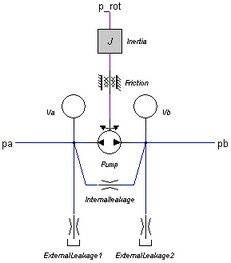﻿ 20-sim webhelp > Library > Iconic Diagrams > Hydraulics > Pumps > displacementpump-leakage

# displacementpump-leakage

## Library

Iconic Diagrams\Hydraulics\Pumps

## Use

Domains: Continuous. Size: 1-D. Kind: Iconic Diagrams (Hydraulics).Description

This model describes a pump with internal and external leakage and a displacement that is proportional to the speed of the input axis:

i = D * c / (2*pi);

pa.phi = pb.phi = i * p_rot.omega;

The actual flows at the inlet and outlet port may be slightly different because of the flow into the lumped volumes and the leakage flows. The leakage flows are modeled by laminar resistances. The torque of the pump is equal to:

p.T = i*(pa.p - pb.p);

If the inlet pressure is smaller than the vapour pressure(p < p_vapour), the flow is zero. If the inlet pressure becomes larger than the vapour pressure the flow gradually grows to its normal value, until the atmospheric pressure (p = 0) is reached.

## Interface

 Ports Description pa pb p_rot inlet port (hydraulic) outlet port (hydraulic) axis (rotation) Causality preferred pressure out pa preferred pressure out pa preferred angular velocity out p_rot Parameters ExternalLeakage1\p_preload ExternalLeakage2\p_preload Va\V Va\B Va\p_initial Vb\V Vb\B Vb\p_initial Pump\D Friction\d Inertia\J Tank pressure [Pa] Conductance of laminar resistance [m3/s.Pa] Tank pressure [Pa] Conductance of laminar resistance [m3/s.Pa] Conductance of laminar resistance [m3/s.Pa] Volume of oil under pressure [m3] Effective bulk modulus [Pa] The starting pressure of the volume [Pa] Volume of oil under pressure [m3] Effective bulk modulus [Pa] The starting pressure of the volume [Pa] Displacement per revolution [m3] Damping [N.m.s/rad] Moment of inertia [kg.m2]